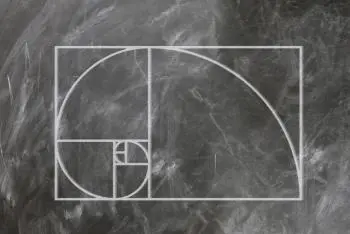# Euclidean Geometry and the Five Fundamental PostulatesEuclidean geometry, named after the ancient Greek mathematician Euclid, has been a fundamental pillar in the world of mathematics since its conception around 300 BC.

His legacy has endured through the centuries, and his influence can be seen in various disciplines, from physics to engineering.

Euclidean geometry is based on the "Elements of Euclid", a work consisting of thirteen books that address various aspects of geometry. In these books, Euclid presents a series of definitions, axioms and postulates that serve as foundations for the study of the properties of space and figures.

One of the distinctive elements of Euclidean geometry is its focus on logical deduction, where each result is derived from previous propositions.

## The Five Fundamental PostulatesEuclidean geometry is based on five fundamental postulates that serve as foundations for the study of the properties of space and geometric figures.

The five postulates are described below:

### Straight Line Postulate

“Given any pair of points, it is possible to draw a single straight line joining them.”

This postulate establishes the existence of a direct connection between two points through a straight line. It is the basis for the notion of direct connection and the shortest distance between two points in Euclidean geometry.

### Infinite Extension Postulate

“A finite straight line can extend indefinitely in both directions.”

This postulate suggests that there are no limits to the length of a straight line. It implies that a straight line can be extended infinitely in both directions, without finding an end.

### Circle Postulate

“Given a center and a radius, it is possible to draw a single circle.”

This postulate allows the construction of circles with any radius and center. A circle is defined as the set of points equidistant from a central point.

### Parallel Postulate

“Given a straight line and a point exterior to it, there is exactly one parallel straight line that passes through the exterior point.”

This postulate has been the subject of debate throughout history and is known as Euclid's parallel postulate. It has led to the development of non-Euclidean geometries, which explore the implications of altering this postulate.

### Angle Postulate

“The sum of the interior angles of a triangle is equal to two right angles (180 degrees).”

This postulate establishes the relationship between the interior angles of a triangle and the total measure of the angles. It is essential for the congruence and similarity of triangles in Euclidean geometry.

## Practical Applications

Euclidean Geometry is not only a set of abstract theorems, but has also found practical applications in various fields.

Architecture, for example, has used Euclidean geometric principles in the design of structures since ancient times. Engineering and classical physics also rely on Euclidean geometry to model the physical world precisely.

### Influence on ArchitectureEuclidean geometry has been the backbone of architecture throughout history, influencing the design and construction of iconic structures. The golden ratio, derived from Euclidean principles, has guided the harmonious arrangement of elements in buildings such as the Parthenon and Notre-Dame Cathedral.

Basic geometric shapes, such as triangles and circles, have been the basis for the design of architectural plans, from the pyramid of Cheops to the dome of St. Peter's Basilica.

Additionally, Euclidean trigonometry has been applied to calculate distances and angles, ensuring precision in construction. The Pythagorean theorem has been essential to guarantee structural stability in the arrangement of columns and walls.

On the other hand, descriptive geometry, derived from Euclid, has allowed projects to be represented in two-dimensional planes, facilitating visual communication in architectural design.

## Later Developments

Despite its wide applicability, Euclidean Geometry has been the subject of criticism and subsequent developments. In the late 19th century, mathematicians such as Nikolai Lobachevsky and János Bolyai explored non-Euclidean geometries, where Euclid's fifth postulate was not valid.

This led to the formulation of hyperbolic geometry and the discovery that there are coherent mathematical worlds in which the angles of a triangle can add up to less than or more than two right angles.

Author:

Published: November 14, 2023
Last review: November 14, 2023